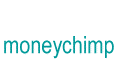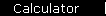# Bond Yield-to-Maturity

Imagine you are interested in buying a bond, at a market price that's different from the bond's par value. There are three numbers commonly used to measure the annual rate of return you are getting on your investment:

 Coupon Rate: Annual payout as a percentage of the bond's par value Current Yield: Annual payout as a percentage of the current market price you'll actually pay Yield-to-Maturity: Composite rate of return off all payouts, coupon and capital gain (or loss)

(The capital gain or loss is the difference between par value and the price you actually pay.)

The yield-to-maturity is the best measure of the return rate, since it includes all aspects of your investment. To calculate it, we need to satisfy the same condition as with all composite payouts:

Whatever r is, if you use it to calculate the present values of all payouts and then add up these present values, the sum will equal your initial investment.

In an equation,

 1. c(1 + r)-1 + c(1 + r)-2 + . . . + c(1 + r)-Y + B(1 + r)-Y = P where c = annual coupon payment (in dollars, not a percent) Y = number of years to maturity B = par value P = purchase price

You should try to form a mental picture of what this equation is saying. The left side represents Y+1 different compound interest curves, all starting out now, and each one ending at the moment that the payout it corresponds to takes place. Most of these curves will lie pretty low to the axis, because they only grow to a value of c, the coupon payment. The very last curve will be a lot taller, and end up at the par value B. And if you add up the present values of all these curves (that's the left side of the equation), the sum will exactly equal the purchase price of the bond (that's the right side).

As with most composite payout problems, equation 1 can't be solved exactly, in general. The nice part is that all yield-to-maturity problems have basically the same form, so people have been able to create programmable calculators and computer programs (and even tables back in the old days) to help you find r.

Example:   Suppose your bond is selling for \$950, and has a coupon rate of 7%; it matures in 4 years, and the par value is \$1000. What is the YTM?

The coupon payment is \$70 (that's 7% of \$1000), so the equation to satisfy is

 2 70(1 + r)-1 + 70(1 + r)-2 + 70(1 + r)-3 + 70(1 + r)-4 + 1000(1 + r)-4 = 950

Of course you aren't really going to solve this, so you just use the popup calculator instead, and find that r is 8.53%. If you want, you can plug this number back into equation 2, just to make sure it checks out.

One thing to notice is that the YTM is greater than the current yield, which in turn is greater than the coupon rate. (Current yield is \$70/\$950 = 7.37%). This will always be true for a bond selling at a discount. In fact, you will always have this:

 Bond Selling At . . . Satisfies This Condition Discount Coupon Rate < Current Yield < YTM Premium Coupon Rate > Current Yield > YTM Par Value Coupon Rate = Current Yield = YTM

## Bond Yields and Prices

Once a bond has been issued and it's trading in the bond market, all of its future payouts are determined, and the only thing that varies is its asking price. If you buy such a bond the yield to maturity you'll get on your investment naturally increases if you can buy it at a lower price: as they say, bond prices and yields "move" in opposite directions. That can be confusing since people aren't always consistent in the way they talk about bond performance. If somebody says "10 year treasuries were down today", they probably mean that the asking price was down (so it was a bad day for bond holders); but they sometimes mean that the yield to maturity was down because the asking price was up (a good day for bond holders).

home  |  article  |  glossary  |  calculator  |  about us  |  booksIntroductionCompound InterestMore CompoundingPresent Value / CAGRComposite InvestmentsBond YieldGeometric SeriesGrowth + ContributionsAnnuityMortgageStock ValuationBooks & Links

 Also see the bond yield calculator.